BIOCHEMISTRY ONLINE D: Thermodynamics - A ReviewHome CHAPTER 1: LIPIDS CHAPTER 2: PROTEINS CHAPTER 3: CARBOHYDRATES CHAPTER 4 - NUCLEIC ACIDS CHAPTER 5: BINDING CHAPTER 6 - TRANSPORT AND KINETICS CHAPTER 7 - CATALYSIS CHAPTER 8: OXIDATIVE-PHOSPHORYLATION CHAPTER 9 - SIGNAL TRANSDUCTION Chapter 10: METABOLIC PATWAYS Favorite Links Contact MeReactions and Energy Change

Why do reactions vary in extent from completely irreversible in the forward reaction to reversible reactions favoring the reactants? It might help to understand a simple physical reaction before we try more complicated chemical reactions.

Ball on a hill. Does a ball at the top of a hill roll downhill spontaneously, or does the opposite happen? No one has ever seen a ball roll spontaneously uphill unless a lot of energy was added to the ball. This physical reaction appears to be irreversible, and occurs because the ball has lower potential energy at the bottom of the hill than it does at the top. The gap in the potential energy is related to the "extent" and spontaneity of this reaction. As we have observed before, nature tends to go to a lower energy state.   By analogy, we will consider the driving force for a chemical reaction to be the free energy difference, DG, between reactants and products.  DG determines the extent and spontaneity of the reaction.

Reversible/Irreversible Reactions, Extent of Reactions, Equilibrium: Consider a hypothetical reversible reaction in which you start with some reactants, A and B, each at a 1 M concentration (1 mol of each/L solution). but no products, P and Q. For ease assume that the total volume of solution is 1 L, so that we start with 1 mol each of A and B . At time t=0, the concentration of products is 0. The reaction can be written as:

A + B <--> P + Q.

As time progresses, the amounts or concentrations of A and B decrease as the amounts or concentrations of products P and Q increase. At some time, no further changes occur in the amount or concentrations of remaining reactants or products. At this point the reaction is in equilibrium, a term used often in our common vocabulary to denote a system that is undergoing no net change.

Most of the reactions that we will study occur in solution, so we will deal with concentrations (in mol/L or mmol/mL = M).   Lets consider how the concentration of reactants and products change as a function of time. Depending on the extent to which a reaction is reversible, 4 different scenarios can be imagined:

Scenario 1: Irreversible reaction in which the reverse reaction occurs to a negligible extent.

In this reaction, the reverse reaction occurs to such a small extent that we can neglect it. The only reaction that occurs is the conversion of reactants to product. Hence all the reactants are converted to product. At equilibrium [A] = 0. Since 1 mol of A reacted, it must form 1 mol of P and 1 mol Q - i.e. the concentration of products at equilibrium is 1 M. At an earlier time of the reaction, (let's pick a time when [A] = 0.8 M), only part of the reactants have reacted (in this case 0.2 M), producing an equal amount of products, P and Q. Graphs of [A] and [P] as a function of timer are shown below.  [A] decreases in a nonlinear fashion to 0 M while [P] increases in a reciprocal fashion to 1 M concentration. This is illustrated in the graph below.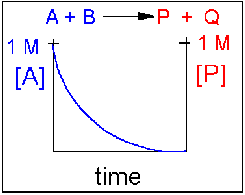Examples of irreversible reactions are reactions of strong acids (nitric, sulfuric, hydrochloric) with bases (OH- and water), or combustion reactions like the burning of sugars (like trees) and hydrocarbons (like octane) to form CO2 and H2O.

Scenario 2: Reversible reaction in which the forward reaction is favored.

Again [A] decreases and [P] increases, but in this case, some A remains since the reaction is reversible. As [A ]and [B] decrease, [P] and [Q] increase, which increases the chance that they will collide and form product. Since P and Q can react to from reactants, the [A] at equilibrium is not zero as is show below.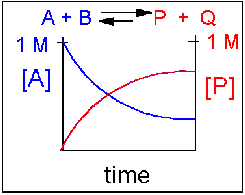Scenario 3: Reversible Reaction in which forward and reverse reactions are equally favored.

Again [A] decreases and [P] increases, but in this case, some A remains since the reaction is reversible. As [A] and [B] decrease, [P] and [Q] increase, which increases the chance that they will collide and form product. Since P and Q can react to from reactants, the [A] at equilibrium is not zero as is show below. Because the reactants and products are equally favored, their concentration will be equal at equilibrium .Scenario 4: Reversible Reaction in which the reverse reaction is favored.

Again [A] decreases and [P] increases, but in this case, some A remains since the reaction is reversible. As [A] and [B] decrease, [P] and [Q] increase, which increases the chance that they will collide and form product. Since P and Q can react to from reactants, the [A] at equilibrium is not zero as is show below. Because the reaction favors reactants. their concentration will be higher at equilibrium than the products.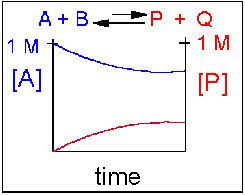An example of this kind of reaction, one that favors reactants, is the reaction of acetic acid (a weak acid) with water.

CH3CO2H(aq) + H2O(l) <==> + H3O+(aq) + CH3CO2-(aq)

Equilibrium Constants:

Without a lot of experience in chemistry, it is difficult to just look at the reactants and products and determine whether the reaction is irreversible, or reversible, favoring either reactants or products (with the exception of obvious irreversible reactions described above). However this data can be found in tables of equilibrium constants.  The equilibrium constant, as its name implies, is constant, independent of the concentration of the reactants and products. A Keq > 1 implies that the products are favored. A Keq < 1 implies that reactants are favored. When Keq = 1, both reactants and products are equally favored. For the more general reaction,

aA + bB <==> pP + qQ,

where a, b, c, and d are the stoichiometric coefficients, Keq = ([P]p[Q]q)/([A]a[B]b), where all the concentrations are those at equilibrium. For a simple reaction where a, b, p, and q are all 1, then Keq = ([P]eq[Q]eq)/([A]eq[B]eq).

(Note:  Equilibrium constants are truly constant only at a given temperature, pressure, and solvent condition.  Likewise, they depend on concentration to the extent that their activities change with concentration.)

For a irreversible reaction, such as the reaction of a 0.1 M HCl(aq) in water, [HCl]eq = 0, so you can't easily measure a Keq. However, if we assume the reaction goes in reverse to an almost imperceptible degree, [HCl]eq might equal 10-10 M. Hence Keq >> 1.

In summary, the extent of reactions can vary from completely irreversible (favoring only the products) to reactions that favor the reactants . Our next goal is to understand what controls the extent of a reaction. Our answer will be change in the Gibbs free energy.  Two different pairs of factors influence the DG.  One pair is concentration and inherent reactivity of reactants compared to products (as reflected in the Keq).  The other pair is enthalpy/entropy changes.  We will now consider the first pair  .

Contributions of Molecule Stability (Keq) and concentration to  DG

Consider the reactions of hydrochloric acid and acetic acid with water.

• HCl(aq) + H2O(l) --> H3O+(aq) + Cl-(aq)
• CH3CO2H(aq) + H2O(l) --> H3O+(aq) + CH3CO2-(aq)

Assume that at t = 0, each acid is placed into water at a concentration of 0.1 M. When equilibrium is reached, there is essentially no HCl left in solution, while 99% of the acetic acid remains. Why are they so different? We rationalized that HCl(aq) is a much stronger acid than H3O+(aq) which itself is a much stronger acid than CH3CO2H(aq). Why? All we can say is there is something about the structure of these acids (and the bases) that makes HCl much more intrinsically unstable, much higher in energy, and hence much more reactive than the acid it forms, H3O+(aq). Likewise, H3O+(aq) is much more intrinsically unstable, much higher in energy, and hence more reactive than CH3CO2H(aq). This has nothing to do with concentration, since the initial concentration of both HCl(aq) and CH3CO2H(aq) were identical. This observation is reflected in the Keq for these acids (>>1 for HCl and <<1 for acetic acid). This difference in intrinsic stability of reactants compared to products (which is independent of concentration) is one factor that contributes to DG.

The other factor is concentration.  A 0.25 M (0.25 mol/L or 0.25 mmol/ml) solution of acetic acid does not conduct electricity, implying that very few ions of H3O+(aq) + CH3CO2-(aq) exist in solution. However, if more concentrated acetic acid is added, a dim light becomes  evident. Adding more reactant seemed to drive the reaction to form more products, even though the reverse reaction is favored if one considers only the intrinsic stability of reactants and products. Before the concentrated acid was added, the system was at equilibrium. Adding concentrated acid perturbed the equilibrium, which drove the reaction to form additional products. This is an example of Le Chatelier's Principle, which states that if a reaction at equilibrium is perturbed, the reaction will be driven in the direction that will relieve the perturbation. Hence:

• if more reactant is added, the rx. shifts to form more products
• if more product is added, the rx. shifts to form more reactants
• if products are selectively removed (by distillation, crystallization, or further reaction to produce another species), the rx. shifts to form more product.
• if reactants are removed (as above), the rx. shifts to form more reactants.
• if heat is added to an exothermic reaction, the rx. shifts to get rid of the excess heat by shifting to form more reactants. (opposite for an endothermic rx).

The total DG can be expressed as the sum of the two contributions showing the effects of the intrinsic stability (Keq) and concentration:

DG = DGstab + DGconc

DG = DGo + RTlnQrx = DGo + RTln ([P][Q])/([A][B])

for the reaction A + B <=> P + Q, where DGo reflects the contribution from the relative intrinsic stability of reactants and products) and RTlnQrx reflects the contribution from the relative concentrations of reactants and products (which has nothing to do with stability).   Qrx is the reaction quotient which for the reaction A + B <=> P + Q is given by:

Qrx   = ([P][Q])/([A][B])

Meaning of DG

Remember that DG is the "driving" force for a reaction, analogous to the difference in potential energy for a ball on a hill. Go back to that analogy. if the ball starts at the top of the hill, does it roll down hill? Of course. It goes from high potential energy to low potential energy. The reaction can be written as: Balltop --> Ballbottom for which the change in potential energy, DPE = PEbottom -PEtop< 0. If the ball starts at the bottom, will it go to the top? Obviously not. For that reaction, Ballbottom --> Balltop, DPE > 0. If the top of the hill was at the same height at the bottom of the hill (obviously an absurd situation), the ball would not move. It would effectively be at equilibrium, a state of no change. For this reaction, Balltop --> Ballbottom, the DPE = 0. As the ball starts rolling down the hill, its potential energy gets closer to the potential it would have at the bottom. Hence the DPE changes from negative to more and more positive until it gets to the bottom at which case the DPE = 0 and movement ceases. If the DPE is not 0, the ball will move until the DPE = 0.

Likewise, for a chemical reaction that favors products, DG < 0. The system is not at equilibrium and the reaction will go in the direction of products. As the reaction proceeds, products build up, and there is less of a driving force for reactants to go to products (LeChatilier's Principle), so the DG becomes more a more positive until the DG = 0 and the reaction is at equilibrium. A reaction that has a DG > 0 is likewise not at equilibrium so it will go in the appropriate direction until equilibrium is reached. Hence for the reaction A + B <==> P + Q,

• if DG < 0, the reaction goes toward products P and Q
• if DG = 0, the reaction is at equilibrium and no further change occurs in the concentration of reactants and products.
• if DG > 0, the reaction goes toward reactants A and B.

We can not measure easily the actual free energy G of reactants or products, but we can measure DG readily. These points are illustrated in the graph below of DG vs time for the hypothetical reaction A + B <==> P + Q. (Also notice the two insert graphs - in blue and red - which show, in analogy to the ball on the hill graphs, the values of DG at the two points where the perturbation to the equilibrium were made.)Notice the DG is constantly changing until the system reaches equilibrium. Initially the equilibrium is perturbed so that the system is not in equilibrium (shown in blue). The perturbation was such that the products are favored. After equilibrium was reached, the system was perturbed again, this time in a fashion to favor the reverse reaction. Notice in this case the DG for the reaction as written: A + B <==> P + Q is positive - i.e. it is not in equilibrium. Therefore the reaction (as written) goes backwards to products. It is important to realize that the reported DG is for the reaction as written.

Now let's apply DG = DGo + RTln Q =  DGo + RTln ([P][Q])/([A][B]) to two reactions we discussed above:

• HCl(aq) + H2O(l) <==>H3O+(aq) + Cl-(aq)
• CH3CO2H(aq) + H2O(l) <==> H3O+(aq) + CH3CO2-(aq)

Assume that at time t=0, 0.1 mole of HCl and CH3CO2H were added to two different beakers. At this point the forward reaction are favored, but obviously to different extents. The RTln Q would be identical for both acids, since each reactant is present at 0.1 M, but no products yet exist. However, the DGo is  negative for HCl and positive for  acetic acid since HCl is a strong acid. Hence at t=0, DG for the HCl reaction is much more negative than for acetic acid. This is summarized in table below. The direction of the arrow shows if products (-->) or reactants (<---) are favored. The size of the arrow shows very approximately to what extent the DG term is favored

 Reaction at t=0 DGo RTln Q DG HCl(aq) + H2O(l) ---------------> ---------------> -----------------------------> CH3CO2H(aq) + H2O(l) <------------- ---------------> ->

Now when equilibrium is reached, no net change occurs in the concentration of reactants and products, and  DG = 0. In the case of HCl, there is just an infinitesimal amount of HCl left, and 0.1 M of each product, so concentration favors HCl formation. However, the intrinsic relative stability of reactants and products still favors products. In the case of acetic acid, most of the acetic acid remains (0.099 M) with little product (0.001 M) so concentration favors product. However, the intrinsic relative stability of reactants and products still favors reactants. This is summarized in table below.

 Reaction at equlib. DGostab RTln Q DG HCl(aq) + H2O(l) ---------------> <--------------- favors neither, = 0 CH3CO2H(aq) + H2O(l) <------------- --------------> favors neither, = 0

Compare the two tables above (one at time t= 0 and the other at equilibrium). Notice:

• DGo never changes, since it has nothing to do with concentration.
• Only RTln Q changes during the course of a reaction, until equilibrium is achieved.

Meaning of DGo

To get a better meaning of the significance of DGo , let's consider the following equations under two different conditions:

DG = DGo + RTln Q =  DGo + RTln ([P][Q])/([A][B])

Condition I: Reaction at equilibrium, DG = 0

The equation reduces to:  DGo =  - RTln ([P]eq[Q]eq)/([A]eq[B]eq) or  DGo =  - RTln Keq  =  - 2.303RTlog Keq

This supports our idea that DGo is independent of concentration since Keq should also be independent of concentration.

Condition II: Concentration of all reactants and products is 1 M (standard state, assuming solution reaction)

The equation reduces to:  DG = DGo + RTln ()/()  = DGo + 2.303RTlog 1 =  DGo

The implies that when all reactants are at this concentration, defined as the standard state (1 M for solutes), the DG at that particular moment just happens to be the DGo for the reaction.  If one of the reactant or products is H3O+, it would make little biological sense to calculate DGo for the reaction using the standard state of [H3O+] = 1 M, or a pH of -1.  Instead, it is assumed the pH = 7, [H3O+] = 10-7 M.  A new symbol is used for  DGo under these condition,  DGo'.

Dependence of the Free Energy on Enthalpy and Entropy

H + H --> H2 Does this reaction occur spontaneously? It does. You should remember that individual H atoms are unstable, since they don't have an completed outer shell of electrons - in this case a duet. As they approach, they can interact to form a covalent bond and in the process release energy. The bonded state is a lower energy state than two separated H atoms. This should be clear since energy has to be added to a molecule of H2 to break the bond.

`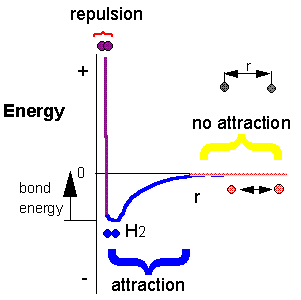2C8H18(l) + 25O2(g) --> 16CO2(g) + 18H2O(g). To carry out this reaction, every C-C, C-H and O-O bond in the reactants must be broken (which requires an input of energy) but a lots of energy is released on formation of C-O and H-O covalent bonds in the products. Is more energy needed to break the bonds in the reactants or is more energy released on formation of bonds in the product? The answer should be clear. The products must be at a lower energy than the reactants since huge amounts of heat and light energy are released on combustion of gasoline and other hydrocarbons.

These reactions suggest that energy must be released for a reaction to proceed to any extent in a given direction.

Now consider, however, the following reaction:

Ba(OH)2.8H2O(s) + 2NH4SCN(s) --> 10H2O(l) + 2NH3(g) + Ba(SCN)2(aq+s)

When these two solids are mixed, and stirred, a reaction clearly takes places, as evidenced by the formation of a liquid (water) and the smell of ammonia. What is surprising is that heat is not released into the surroundings in this reaction. Rather heat was absorbed from the surroundings turning the beaker so cold that it freezes to a piece of wood (with a layer of water added to the wood) on which it was placed. This reaction seems to violate our idea that a reaction proceeds in a direction in which heat is liberated. Reactions which liberate heat and raise the temperature of the surroundings are called exothermic reactions. Reactions which absorb heat from the surroundings and hence lower the temperature of the surroundings are endothermic reactions.

The System, Surroundings, and the Universe: First and Second Laws of Thermodynamics

You may remember from General Chemistry that the change in the internal energy of a system, DE, is given by:

DEsys = q + w = q - PextΔV

where q is the heat (thermal energy) transferred to the system (+), from the system (-), w is the work done on (+) or by (-) the system.  If only PV work is done, w = - PextΔV, where Pext is the external pressure resisting a volume change in the system, ΔV.  When pressure is constant, and only PV work is done,

qp = DEsys +  PextΔV = ΔH,

where qp is the heat transferred at constant P (easily measured in a coffee cup calorimeter) which is equal to the change in enthalpy, ΔH, of the system.  For exothermic reactions, the reactants have more thermal energy than the products, and the heat energy (measured in kilocalories) released is the difference between the energy of the products and reactants. When heat energy is used to measure the difference in energy, we call the energy enthalpy (H) and the heat released as the change in enthalpy (DH), as illustrated below.For exothermic reactions, DH < 0. For endothermic reactions, DH > 0.

The equation DEsys = q + w = q - PextΔV  is  one expression of the First Law of Thermodynamics.  Another, a statement of energy conservation, is:

DEtotDEuniverseDEsysDEsurr.= 0.

Clearly, there must be something more that decides whether a reaction goes to a significant extent other that if heat is released from the system.  That is, the spontaneity of a reaction must depend on more than just  ΔHsys.  . Another example of a spontaneous natural reaction is the evaporation of water (a physical, not chemical process).

H2O (l) --> H2O (g)

Heat is absorbed from the surroundings to break the intermolecular forces (H bonds) among the water molecules (the system), allowing the liquid to be turned into a gas. If the surroundings are the skin, evaporation of water in the form of sweat cools the body. What are these reactions spontaneous and essentially irreversible even though they are endothermic? Notice that in both of these endothermic reactions (the reactions of Ba(OH)2.8H2O(s) and 2NH4SCN(s) and the evaporation of water), the products are more disorganized (more disordered) than the products. A solid is more ordered than a liquid or gas, and a liquid is more ordered than a gas.  In nature, ordered things become more disordered with time. Entropy (S), the other factor (in addition to enthalpy changes) is often considered to be a measure of the disorder of a system. The greater the entropy, the greater the disorder.  For reactions that go from order (low S) to disorder (high S), the changed in S, DS > 0. For reaction that goes from low order to high order, DS < 0.

However, this common description of entropy is quite misleading.  Macroscopic examples describing order/disordered states (such as the cleanliness of your room or the shuffling of a deck of card) are inappropriate since entropy deals with microscopic states.

The driving force for spontaneous reactions is the dispersion of energy and matter.  Increases in entropy for reactions that involve matter occur when gases or solutes in solution are dispersed, leading to increases in positional entropy.  For reactions involving energy changes, entropy increases when energy is dispersed as random, undirected thermal motion, leading to increases in thermal entropy.  In this sense, entropy, S (a measure of

("spreadedness") is a measure of number of different ways (microstates) that particles or energy can be arranged (W), not a measure of disorder!  W is an abbreviation for the German word, Wahrscheinlichkeith, which means probabilty.  It can be shown that for a solute dissolving in a solvent,  Wsys = Wsolute x Wsolvent.  Note that this is a multiplicative function.  Entropy is a logarithmic function of W which allows additivity of solute and solvent  W values, a feature found in other thermodynamic state functions like ΔE,  ΔH, and ΔS.  Hence ln Wsys = ln Wsolute + ln WsolventBoltzman showed that

S = k ln W  where k is the Boltzman constant (1.68 x 10-23 J/K), S units: J/K
for molecules,  or

S = kNA ln W = RlnW (J/K.mol) for moles of molecules.

Boltzman realized the connection between the macroscopic entropy of a system and the microscopic order/disorder of a system through the equation S = klnW,   Increasing S (macroscopic property) occurs with increasing numbers of possible microscopic states for the atoms and molecules of a system.

The dissolution of a solute in water and the expansion of a gas into a vacuum, both which proceed spontaneously toward an increase in matter dispersal, are examples of familiar processes characterized by a ΔSsys > 0.

The spontaneity of exothermic and endothermic processes will depend on the  DStotDSsurr +  DSsys.  DSsys often depends on matter dispersal (positional entropy).   DSsurr depends on energy changes in the surroundings, ΔHsurr = -ΔHsys (thermal entropy).

It is more convenient to express thermodynamic properties based on the system which is being studied, not on the surrounding.  This can be readily done for the ΔSsurr which depends both on ΔHsys and the temperature.  First consider the dependency on  ΔHsys.  thermal energy flow into or out of the system, and since ΔHsys = - ΔHsurr,

ΔSsurr a -ΔHsys

For an exothermic reaction,  ΔSsurr > 0 (since ΔHsys < 0) and the reaction is favored;

For an endothermic reaction, ΔSsurr < 0, (since ΔHsys > 0), and the reaction is disfavored;

DSsurr  also depends on the temperature T of the surroundings:

ΔSsurr a 1/T

If the Tsurr is high, a given heat transfer to or from the surroundings will have a smaller effect on the ΔSsurr; conversely, if the Tsurr is low, the effect on ΔSsurr will be greater.  (Atkins, in his recent General Chemistry,  uses the analogy of the effect of a sneeze in library compared to in a crowded street;   The new American Chemistry General Chemistry text uses the analogy of giving \$5 to a friend with \$1000 compared to one who has just \$10.)  Hence,

DSsurr = -ΔHsys/T  (Note: from a more rigorous thermodynamic approach, entropy can be determined from dS = dqrev/T.)

DStot  =  DSsurr  +  DSsys

ΔStot depends on both enthalpy changes in the system and entropy changes in the surroundings.

DStot   =  -  DHsys/T +  DSsys        Multiplying both sides by -T gives

-TΔStot =   ΔHsys  TΔSsys

The thermodynamic function Gibb's Free Energy, G, can be defined as:  G = H - TS;

At constant T and P,  ΔG = ΔH - TΔS

Hence

ΔGsys = ΔHsys -  TΔSsys  = - TΔStot

Spontaneity is determined by ΔStot OR  ΔGsys  since ΔStot = -ΔGsys/T .    ΔGsys is widely use in discussing spontaneity since it is a state function, depends only on the enthalpy and entropy changes in the system, and is negative (as is the potential energy change for a falling object) for all spontaneous processes.

The second law of thermodynamics can be succinctly stated:  For any spontaneous process, the ΔStot > 0.   Unlike energy (from the First Law), entropy is not conserved.

Summary:

Whether reactions are spontaneous in a given direction depends not just if the reaction is exothermic (since some reactions are endothermic) or just entropy (since some reactions that increase the number of microstates or energy dispersal occur spontaneously) but on some combination of both, as illustrated in the table below.

 reaction D enthalpy (DH) D entropy (DS) favored/disfavored combustion of fuels exothermic (<0) positive (>0) always favored various exothermic (<0) negative(<0) depends various endothermic (>0) positive (>0) depends synthesis of sugars from CO2 and H2O endothermic (>0) negative (<0) always disfavored

Once again, the total DG is a function of two thermodynamic parameters, DH and DS.  You will remember the following equations from general chemistry:

DG = DH  -  TDS   and  DGo = DHo  -  TDSo

which describes the free energy, enthalpy, and entropy for any condition (DG) and for the standard state (DGo).  Previously, we saw that DG was a function of two other terms that were more directly related to the actual chemistry of the system - the equilibrium constant, Keq, and the concentrations of reactants and products.

DG = DGo + RTlnQ = DGo + RTln ([P][Q])/([A][B])

Summary: DG determines if a reaction goes spontaneously in any given direction. If DG < 0, the reaction goes from reactants to products - to a state when products have a lower energy than reactants - like a ball rolling down a hill. Many factors contribute to DG, including RTln Q,  DGo and DH. These are all energy terms and hence they will favor product formation if they are < 0. The other term which affects DG is DS. Entropy favors a product if DS >0. DS is not an energy term, but notice in the above equation that the term containing the contribution from entropy is -TDS , where T is the temperature. This term is < 0, and favors product, if DS is positive (>0).

Speed of Reactions

One thing that we haven't studied is the speed (not extent) of a reaction. They are two very different concepts. Consider for example the simple reaction of octane with oxygyen.

2C8H18(l) + 25O2(g) --> 16CO2(g) + 18H2O(g).

This reaction is clearly exothermic (DH < 0) and proceeds with an increase in disorder (DS>0). Therefore, it is very favored in the forward direction. But you all know from experience that if you pour gasoline on to a table in the presence of air, nothing seems to happen. How can this be if the reaction is so favorable that it is essentially irreversible? A look at the diagram below shows how we can rationalize this behavior. If you think about it , the first thing that must happen in the reaction above is that some bonds in the products must be broken. This is clearly an endothermic process. Energy (through collisions) must be put into the reactants to do this. The molecules then go to a higher energy state. Eventually a few molecule then start forming stable bonds found in the product and in doing so, they release a lots of energy, in the form of heat. This released heat increases the temperature of the container, causing the molecules to have increased kinetic energy, increasing the number of collisions between reactant molecules which cause a cascade of bond breaking and making, resulting in a huge release of energy. This is why a spark is needed to "get the reaction going", even though it is greatly favored.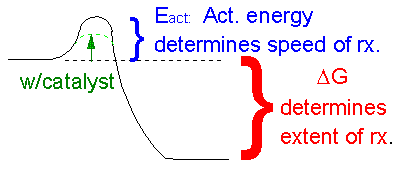Enter supporting content here Biochemistry - Where molecules become life...